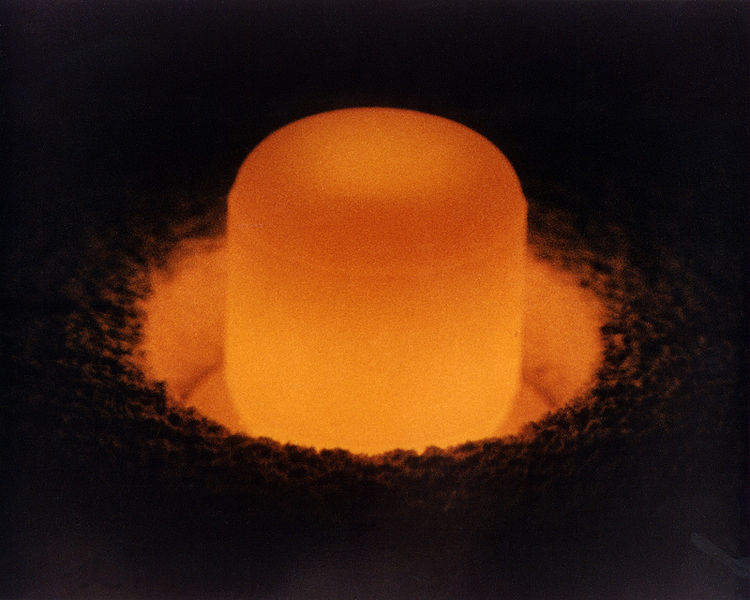# A Radioactive Problem

Chemistry Level 4Plutonium-239 has been used as a power source for heart pacemakers. The chemical reaction taking place is

$_{94}^{239}\ce{Pu} \longrightarrow _{2}^{4}\ce{He} + _{92}^{235}\ce{U}$

Let the energy obtained from the following decay of $215 \ \text{mg}$ of plutonium-239 be $A \ \text{J}$.

Let $B \ \text{g}$ be the minimum mass of $\text{Zn}$ that would be needed for the standard voltaic cell

$\ce{Zn} \ | \ \ce{Zn^{2+}} \ || \ \ce{Cu^{2+}} \ | \ \ce{Cu}$

to obtain the same amount of electrical energy.

What is the value of $\frac{A}{1000B}$ when rounded to the nearest integer?

Details and Assumptions

• Standard cell potential: $E^{\circ}_{\text{cell}} = 1.1 \ \text{V}$.
• Atomic masses: $\ce{He} = 4.0015 \ \text{u}, \ce{U} = 234.9935 \ \text{u}, \ce{Pu} = 239.0006 \ \text{u}$.

#### You can try more of my Questions here.

×

Problem Loading...

Note Loading...

Set Loading...